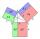# Angles by cosine law

Calculate the size of the angles of the triangle ABC, if it is given by: a = 3 cm; b = 5 cm; c = 7 cm (use the sine and cosine theorem).

Result

A =  21.787 °
B =  38.213 °
C =  120 °

#### Solution:Try calculation via our triangle calculator.Leave us a comment of example and its solution (i.e. if it is still somewhat unclear...):

Showing 0 comments:Be the first to comment!#### To solve this example are needed these knowledge from mathematics:

Cosine rule uses trigonometric SAS triangle calculator. See also our trigonometric triangle calculator. Pythagorean theorem is the base for the right triangle calculator.

## Next similar examples:

1. AnglesIn the triangle ABC, the ratio of angles is: a:b = 4: 5. The angle c is 36°. How big are the angles a, b?
2. In a 2In a thirteen sided polygon, the sum of five angles is 1274°, four of the eight angles remaining are equal and the other four are 18° less than each of the equal angles. Find the angles. .
3. Greatest angleCalculate the greatest triangle angle with sides 197, 208, 299.
4. Side cIn △ABC a=2, b=4 and ∠C=100°. Calculate length of the side c.
5. Triangle and its heightsCalculate the length of the sides of the triangle ABC, if va=5 cm, vb=7 cm and side b is 5 cm shorter than side a.
6. Bearing - navigationA ship travels 84 km on a bearing of 17°, and then travels on a bearing of 107° for 135 km. Find the distance of the end of the trip from the starting point, to the nearest kilometer.
7. The pondWe can see the pond at an angle 65°37'. Its end points are 155 m and 177 m away from the observer. What is the width of the pond?
8. Scalene triangleSolve the triangle: A = 50°, b = 13, c = 6
9. Medians of isosceles triangleThe isosceles triangle has a base ABC |AB| = 16 cm and 10 cm long arm. What are the length of medians?
10. Triangle ABCTriangle ABC has side lengths m-1, m-2, m-3. What has to be m to be triangle a) rectangular b) acute-angled?
11. LawsFrom which law follows directly the validity of Pythagoras' theorem in the right triangle? ?
12. If theIf the tangent of an angle of a right angled triangle is 0.8. Then its longest side is. .. .
13. Triangle ABCIn a triangle ABC with the side BC of length 2 cm The middle point of AB. Points L and M split AC side into three equal lines. KLM is isosceles triangle with a right angle at the point K. Determine the lengths of the sides AB, AC triangle ABC.
14. Reference angleFind the reference angle of each angle:
15. Add vectorGiven that P = (5, 8) and Q = (6, 9), find the component form and magnitude of vector PQ.
16. TrigonometryIs true equality? ?
17. Theorem proveWe want to prove the sentense: If the natural number n is divisible by six, then n is divisible by three. From what assumption we started?